﻿ 弗兰克–赫兹实验灯丝电压对实验结果影响的研究 Study on the Effect of Filament Voltage in the J. Frank-G. Hertz Experiment on the Experimental Results

Optoelectronics
Vol.08 No.03(2018), Article ID:26533,8 pages
10.12677/OE.2018.83012

Study on the Effect of Filament Voltage in the J. Frank-G. Hertz Experiment on the Experimental Results

Gaoyao Wei, Xingyu Bao, Zhong Lu

College of Science, Zhejiang University of Technology, Hangzhou Zhejiang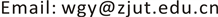Received: Jul. 31st, 2018; accepted: Aug. 15th, 2018; published: Aug. 22nd, 2018ABSTRACT

The influence of the filament voltage variation on the experimental results of J. Frank-G. Hertz Experiment is investigated. The approximate temperature of the filament can be obtained. With the curve IA-UG2K measured, it is found that in the J. Frank-G. Hertz Experiment with the same UK, the maximum positions of the characteristic curve IA-UG2K could be linked together as a straight line, and the intersection of the lines with different filament voltages is just the same as the size of the spurn voltage UG 2A .

Keywords:The J. Frank-G. Hertz Experiment, The Filament Voltage, Least Square Method1. 引言

1913年，丹麦物理学家玻尔(N. Bohr)提出了氢原子的量子模型，指出原子存在能级，原子光谱中的每条谱线来自于原子从某一个较高能态向另一个较低能态跃迁时的辐射，从而成功地解释了氢原子光谱规律。1912~1914年夫兰克和赫兹进行了一系列实验来证明这一理论。他们利用电场使热阴极电子加速获得能量，与管中汞蒸气原子发生碰撞，实验发现电子能量末达到某一临界值时，电子与汞原子发生弹性碰撞，电子不损失能量；当电子能量达到某一临界值时，发生非弹性碰撞，电子的一定能量传递给汞原子，使后者激发，并观察到汞原子跃迁发射的谱线。夫兰克–赫兹实验(简称F-H实验)表明原子的能量只能是一系列离散值，这说明原子的能级的存在，从而直接证明了原子的量子理论的正确性。这一研究成果使夫兰克和赫兹同获1925年诺贝尔物理学奖 。

F-H实验至今仍是探索原子结构的重要手段之一，实验中用的“拒斥电压”筛去小能量电子的方法，己成为广泛应用的实验技术，并已成为一个传统而重要的大学物理实验项目。该实验除了能验证原子能级的存在，还涉及到热电子发射、电子与原子的碰撞过程，以及原子能级跃迁与光的发射、光电效应等物理过程，可以为学生提供多方面的观察与研究。因此，在F-H实验中，影响实验结果的因素十分复杂，影响弗兰克–赫兹法测能级的因素有很多，主要的有灯丝电压、第一栅极电压、拒斥电压、靶原子浓度、弗兰克–赫兹管尺寸、温度等，要得到理想的实验结果，选择合适的工作参量十分重要 。本文主要分析研究灯丝电压对实验结果的影响。

2. 实验装置

3. 灯丝电压对实验的影响

3.1. 实验原理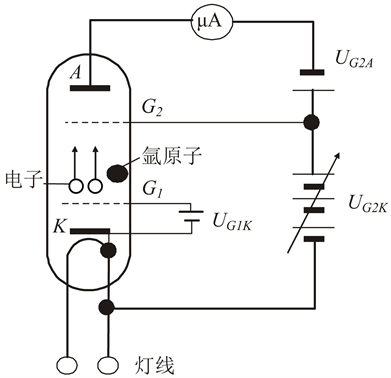Figure 1. F-H diagram

$\frac{\partial f}{\partial t}+\frac{\partial f}{\partial x}v+\frac{\partial f}{\partial v}\frac{F}{m}=0$ (1)

$f=C\mathrm{exp}\left\{-\frac{1}{kT}\left[\frac{1}{2}m{v}^{2}+{E}_{\text{p}}\left(x\right)\right]\right\}$ (2)

$f=C\mathrm{exp}\left\{-\frac{1}{kT}\left[\frac{1}{2}m{v}^{2}-eU\left(x\right)\right]\right\}$ (3)

$\frac{1}{2}m{v}^{2}=e{U}_{\text{K}}$ (4)

$C\underset{{V}_{\text{K}}}{\int }{\text{d}}^{3}x\underset{-\infty }{\overset{+\infty }{\int }}{\text{d}}^{3}v\mathrm{exp}\left\{-\frac{1}{kT}\left[\frac{1}{2}m{v}^{2}-eU\left(x\right)\right]\right\}=1$ (5)

$\frac{{n}_{2}}{{n}_{1}}=\mathrm{exp}\left[\frac{e}{kT}\left({U}_{2}-{U}_{1}\right)\right]$ (6)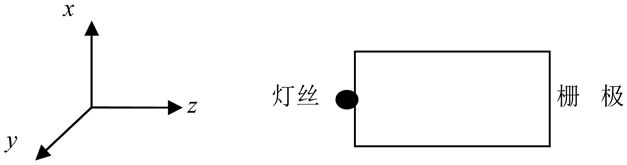Figure 2. Model of filament to grid K

$\frac{{I}_{2}}{{I}_{1}}=\frac{{n}_{2}}{{n}_{1}}=\mathrm{exp}\left[\frac{e}{kT}\left({U}_{2}-{U}_{1}\right)\right]$ (7)

$\mathrm{ln}I=AU+B$ (8)

3.2. 实验条件设置

3.3. 实验结果分析

$\overline{T}=\frac{e}{k\overline{A}}\approx 1755\text{\hspace{0.17em}}\text{K}$ (9)

$\Delta T=|\overline{T}\frac{\Delta A}{\overline{A}}|\approx 30\text{\hspace{0.17em}}\text{K}$ (10)

$T=\overline{T}±\Delta T\approx \left(176±3\right)×10\text{\hspace{0.17em}}\text{K}$ (11)Table 2. The current (×10−7 A) at the minimum values with different filament voltagesTable 3. The current (×10−7 A) at the maximum values with different filament voltages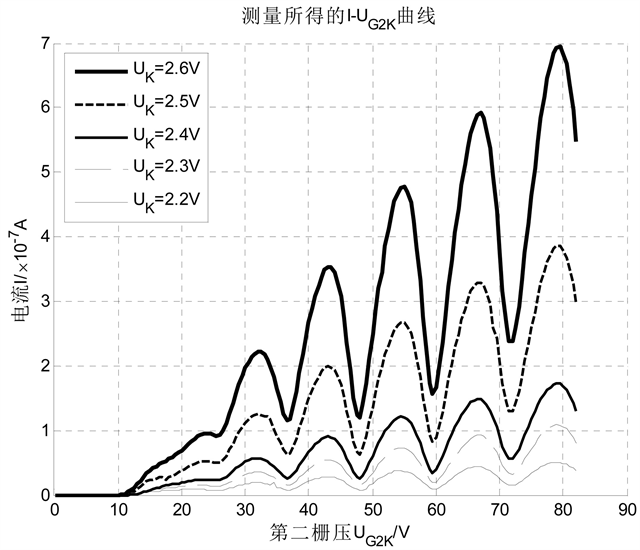Figure 3. Experimental characteristic curve with various filament voltages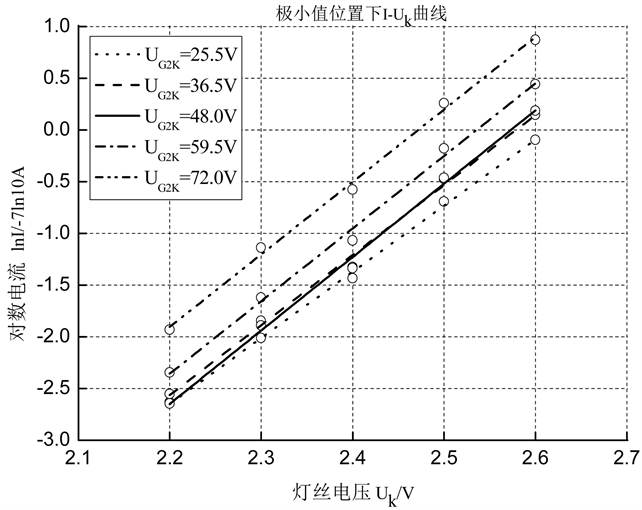Figure 4. The relationship between current and filament voltage at the minimum position

4. 结论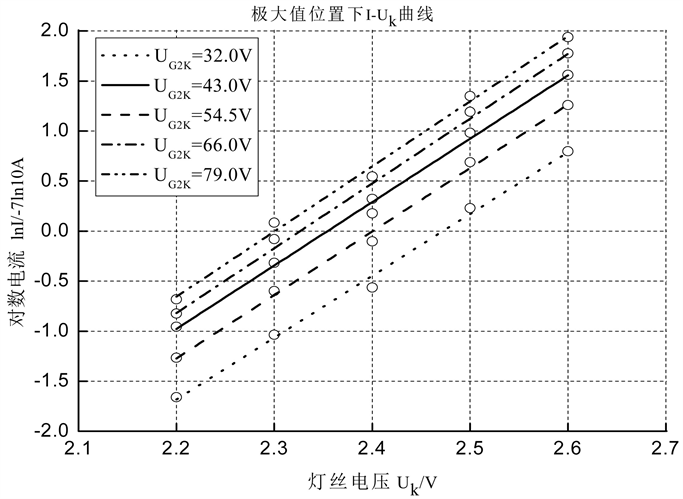Figure 5. The relationship between current and filament voltage at the maximum position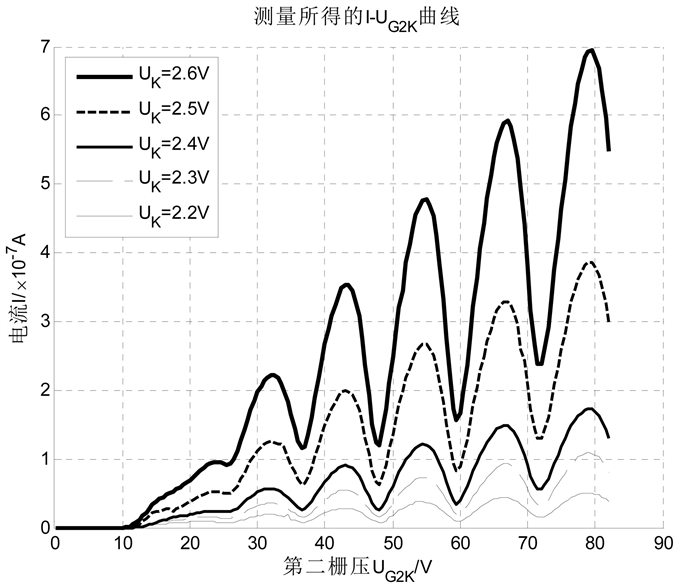Figure 6. Experimental characteristic curve with each filament voltage

① 丝电压UK的增大会使得测量曲线整体的扩大，且检测的电流I随电压呈指数变化，灯丝电压不能过大也不能过小。UK过大会损坏灯丝，过小会使得微安表检测不出。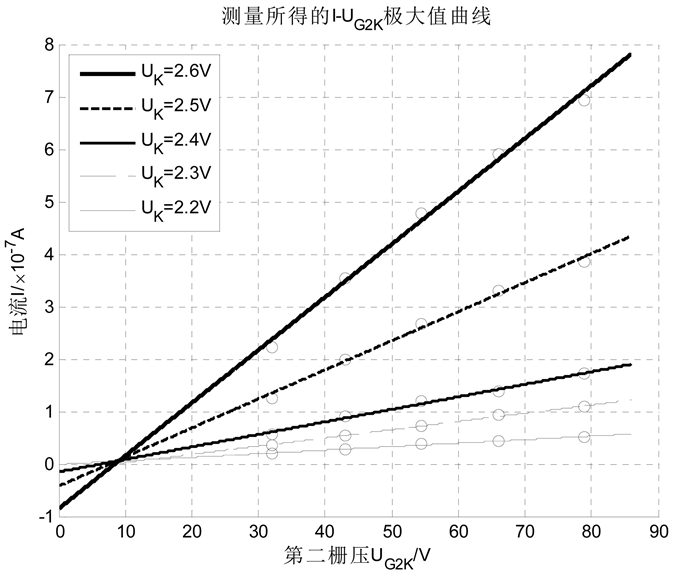Figure 7. The maximum value curves at different filament voltages

② 可以通过检测电流I与灯丝电压UK的关系估计灯丝温度。

③ 通过观察I-UG2K曲线中各个极大值位置可以知道极大值是随UG2K线性增加的。

Study on the Effect of Filament Voltage in the J. Frank-G. Hertz Experiment on the Experimental Results[J]. 光电子, 2018, 08(03): 81-88. https://doi.org/10.12677/OE.2018.83012

1. 1. 隋成华, 魏高尧. 大学物理实验[M]. 北京: 高等教育出版社, 2016: 297-305.

2. 2. 孙桂芳, 阮树仁, 盛淑芳, 钱霞. 夫兰克一赫兹实验中氩原子第一激发电位的研究[J]. 物理实验, 2017(37): 1-3.

3. 3. 陈小凡. 夫兰克一赫兹实验中的伏安特性曲线[J]. 大学物理实验, 2015, 28(5): 80-81.

4. 4. 安可, 高松松, 林上金, 胡澄. 如何选择夫兰克一赫兹实验的工作参量[J]. 大学物理实验, 2014, 27(6): 34-36.

5. 5. 金哲. 夫兰克一赫兹实验最佳条件的确定[J]. 延边大学学报(自然科学版), 2006, 32(3): 169-171.

6. 6. 张里荃, 马艳梅, 郝二娟. 夫兰克一赫兹实验最佳实验条件及第一激发电位的研究[J]. 物理实验, 2011, 31(8): 37-38.

7. 7. W•泡利. 泡利物理学讲义4统计力学[M]. 北京: 人民教育出版社, 1982: 11-13.